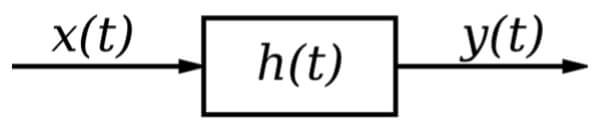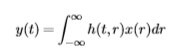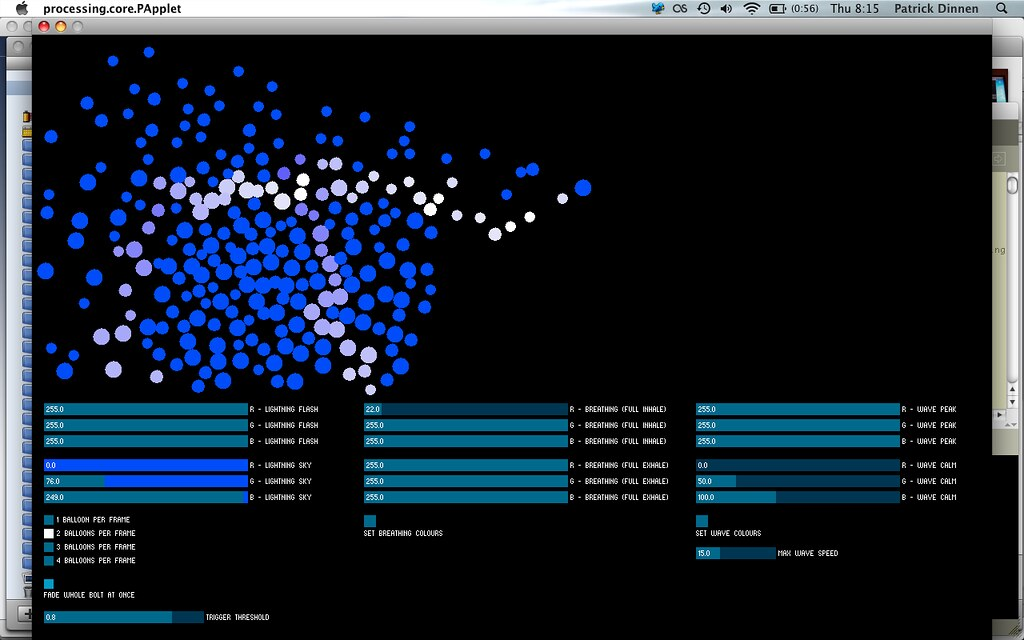# Control Systems Assignment Help

## System Identiﬁcation

### Systems

Systems, in one sense, are devices that take input and produce an output. A system can be thought to operate on the input to produce the output. The output is related to the input by a certain relationship known as the system response. The system response usually can be modeled with a mathematical relationship between the system input and the system output.

### System Properties

Physical systems can be divided up into a number of diﬀerent categories, depending on particular properties that the system exhibits. Some of these system classiﬁcations are very easy to work with and have a large theory base for analysis. Some system classiﬁcations are very complex and have still not been investigated with any degree of success. By properly identifying the properties of a system, certain analysis and design tools can be selected for use with the system.

### Initial Time

The initial time of a system is the time before which there is no input. Typically, the initial time of a system is deﬁned to be zero, which will simplify the analysis signiﬁcantly. Some techniques, such as the Laplace Transform require that the initial time of the system be zero. The initial time of a system is typically denoted by t0. The value of any variable at the initial time t0 will be denoted with a 0 subscript. For instance, the value of variable x at time t0 is given by:

```{`
x(t0)=x0
Likewise, any time t with a positive subscript are points in time after t0, in ascending order:
t0 ≤ t1 ≤t2 ≤···≤ tn
So t1 occurs after t0, and t2 occurs after both points. In a similar fashion above, a variable with a positive subscript (unless specifying an index into a vector) also occurs at that point in time:
x(t1)=x1
x(t2)=x2
This is valid for all points in time t.
`}```

A system satisﬁes the property of additivity, if a sum of inputs results in a sum of outputs. By deﬁnition: an input of x3(t)=x1(t)+x2(t) results in an output of y3(t)=y1(t)+y2(t). To determine whether a system is additive, use the following test: Given a system f that takes an input x and outputs a value y, assume two inputs (x1 and x2) produce two outputs:

```{`
y1 =f(x1)
y2 =f(x2)
Now, create a composite input that is the sum of the previous inputs:
x3 =x1+x2
Then the system is additive if the following equation is true:
y3 =f(x3)=f(x1+x2)=f(x1)+f(x2)=y1+y2
`}```

### Homogeneity

A system satisﬁes the condition of homogeneity if an input scaled by a certain factor produces an output scaled by that same factor. By deﬁnition: an input of ax1 results in an output of ay1. In other words, to see if function f() is homogeneous, perform the following test: Stimulate the system f with an arbitrary input x to produce an output y:

```{`
y =f(x)
Now, create input x1, scale it by a multiplicative factor C(C is an arbitrary constant value), and produce a corresponding output y1:
y1 =f(Cx1)
Now, assign x to be equal to x1:
x1 =x Then, for the system to be homogeneous, the following equation must be true:
y1 =f(Cx)=Cf(x)=Cy
`}```

### Linearity

A system is considered linear if it satisﬁes the conditions of Additivity and Homogeneity. In short, a system is linear if the following is true: Take two arbitrary inputs, and produce two arbitrary outputs:

```{`
y1 =f(x1)
y2 =f(x2) Now, a linear combination of the inputs should produce a linear combination of the outputs:
f(Ax+By) = f(Ax) + f(By) = Af(x) + Bf(y)
This condition of additivity and homogeneity is called superposition. A system is linear if it satisﬁes the condition of superposition.
`}```

### Memory

A system is said to have memory if the output from the system is dependent on past inputs (or future inputs!) to the system. A system is called memoryless if the output is only dependent on the current input. Memoryless systems are easier to work with, but systems with memory are more common in digital signal processing applications. Systems that have memory are called dynamic systems, and systems that do not have memory are static systems.

### Causality

Causality is a property that is very similar to memory. A system is called causal if it is only dependent on past and/or current inputs. A system is called anti-causal if the output of the system is dependent only on future inputs. A system is called non-causal if the output depends on past and/or current and future inputs. A system design that is not causal cannot be physically implemented. If the system can't be built, the design is generally worthless.

### Time-Invariance

A system is called time-invariant if the system relationship between the input and output signals is not dependent on the passage of time. If the input signal x(t) produces an output y(t) then any time shifted input, x(t+δ), results in a time-shifted output y(t+δ) This property can be satisﬁed if the transfer function of the system is not a function of time except expressed by the input and output. If a system is time-invariant then the system block is commutative with an arbitrary delay.

## System Modeling

### The Control Process

It is the job of a control engineer to analyze existing systems, and to design new systems to meet speciﬁc needs. Sometimes new systems need to be designed, but more frequently a controller unit needs to be designed to improve the performance of existing systems. When designing a system, or implementing a controller to augment an existing system, we need to follow some basic steps:

1. Model the system mathematically
2. Analyze the mathematical model
3. Design system/controller
4. Implement system/controller and test

### External Description

An external description of a system relates the system input to the system output without explicitly taking into account the internal workings of the system. The external description of a system is sometimes also referred to as the Input-Output Description of the system, because it only deals with the inputs and the outputs to the systemIf the system can be represented by a mathematical function h(t, r), where t is the time that the output is observed, and r is the time that the input is applied. We can relate the system function h(t, r) to the input x and the output y through the use of an integral:### Email Based Assignment Help in Control Systems

Here we facilitate best online services in assignmenthelp. net on the graduate and engineering level in control system. Here Assignment Help net gives you cheapest, facile and easy to accessing. Assignment Help net services provided all over the world.

You will get all answers of your problems which is in your assignment, homework at our Assignment Help services at any level as school, university students. Finding control system Assignment Help is quickest services, send your assignment to us through an e-mail and also declare date of receiving. As we all know that control Systems is complex subject in engineering, but we will make it easy for you by the help of our expertise. Control Systems Assignment Help also helps students with Control Systems lesson plans and work sheets.